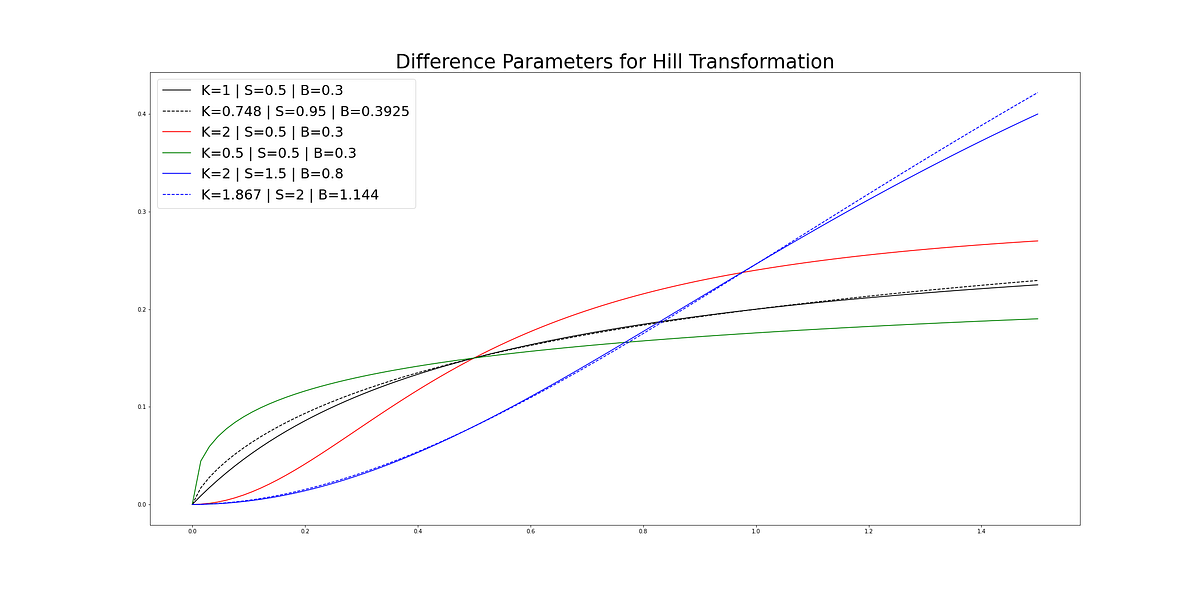# Carryover and Shape Effects in Media Mix Modeling: Paper ReviewIn the following post, I’ll review and implement the main portions of “Bayesian Methods for Media Mix Modeling with Carryover and Shape Effects”.

The full paper can be found here: link

— — —

To begin, media mix models (MMM) aim to uncover the causal effect of paid media on a metric of interest, typically sales. Historically, the problem has largely been modeled via linear regression and the causal impact has been derived using Rubin’s potential outcomes framework.

In simple (data science) terms, this translates to

1. Training a regression model that predicts sales using media spend and control variables.
2. Deriving causal impact by comparing sales when media spend is at observed amount and when it is set to zero.

Estimating casual impact from observational data has a number of issues i.e. “correlation doesn’t equal causation” for starters. And media mix models have a host of unique issues to take note of. An excellent review of these issues can be found here: Challenges And Opportunities In Media Mix Modeling

This paper focuses on two specific issues:

• Carryover Effects i.e. lagged effects
• Shape Effects i.e. diminishing returns

While also providing a Bayesian model, ROAS calculations and optimization methods.

### Carryover Effects

Carryover effects, often called lagged effects, occur when media spend effects sales across a number of days. For example, if we spend \$100 on display advertising today, we may not see the effects of this spend for several days. The adstock function attempts to parameterize this phenomenon and the paper takes two approaches to adstock modeling:

Geometric

• This is a weighted average going back L days, where L can vary by media channel.
• The effect has the largest impact on the day of spend and decays thereafter.

• The effect of media spend spikes T (theta) days after media spend.

### Implementation

``````def geoDecay(alpha, L):
'''
weighted average with geometric decay

weight_T = alpha ^ T-1

returns: weights of length L to calculate weighted averages with.
'''
return alpha**(np.ones(L).cumsum()-1)

'''
weighted average with dealyed adstock function

weight_T =

returns: weights of length L to calculate weighted averages with.
'''
return alpha**((np.ones(L).cumsum()-1)-theta)**2

def carryover(x, alpha, L, theta = None, func='geo'):
'''
1\. x is a vector of media spend going back L timeslots, so it should be len(x) == L

2\. Weights is a vector of length L showing how much previous time periods spend has on current period.

3\. L is max length of Lag.

returns transformed vector of spend

## update with numpy average
## np.average(x[:2], weights=[1,.9])
'''
transformed_x = []
if func=='geo':
weights = geoDecay(alpha, L)

elif func=='delayed':

for t in range(x.shape):
upper_window = t+1
lower_window = max(0,upper_window-L)
current_window_x = x[:upper_window]
t_in_window = len(current_window_x)
if t < L:
new_x = (current_window_x*np.flip(weights[:t_in_window], axis=0)).sum()
transformed_x.append(new_x/weights[:t_in_window].sum())
elif t >= L:
current_window_x = x[upper_window-L:upper_window]
ext_weights = np.flip(weights, axis=0)
new_x = (current_window_x*ext_weights).sum()
transformed_x.append(new_x/ext_weights.sum())

return np.array(transformed_x)
``````

## 50 Data Science Jobs That Opened Just Last Week

Data Science and Analytics market evolves to adapt to the constantly changing economic and business environments. Our latest survey report suggests that as the overall Data Science and Analytics market evolves to adapt to the constantly changing economic and business environments, data scientists and AI practitioners should be aware of the skills and tools that the broader community is working on. A good grip in these skills will further help data science enthusiasts to get the best jobs that various industries in their data science functions are offering.

## Data Science With Python Training | Python Data Science Course | Intellipaat

🔵 Intellipaat Data Science with Python course: https://intellipaat.com/python-for-data-science-training/In this Data Science With Python Training video, you...

## Applications Of Data Science On 3D Imagery Data

The agenda of the talk included an introduction to 3D data, its applications and case studies, 3D data alignment and more.

## Data Science Course in Dallas

Become a data analysis expert using the R programming language in this [data science](https://360digitmg.com/usa/data-science-using-python-and-r-programming-in-dallas "data science") certification training in Dallas, TX. You will master data...

## 32 Data Sets to Uplift your Skills in Data Science | Data Sets

Need a data set to practice with? Data Science Dojo has created an archive of 32 data sets for you to use to practice and improve your skills as a data scientist.